﻿ Multiproticity of Weak Acids: Inflection Point vs. Equivalence Point

Multiproticity of Weak Acids: Inflection Point vs. Equivalence Point

Shazia Perveen, Sheikh Mohiuddin

World Journal of Chemical Education

Multiproticity of Weak Acids: Inflection Point vs. Equivalence Point

Shazia Perveen1,, Sheikh Mohiuddin1

1Department of chemistry, University of Karachi, Karachi, Pakistan

Abstract

Most of the laboratory experiments of undergraduate chemistry curriculum are based on pH metry for qualitative and quantitative analysis. Establishment of any acid’s profile in terms of it being mono, di, or triprotic is a common trend in lab exercises. In these tasks, experiments are designed to classify multiprotic acids in terms of their stepwise proton releasing capability. Strong acid-base pH titration curves have such equivalence and inflection points that could be identified with ease. However, some multiprotic weak acids have such equivalence or inflection points which are difficult to identify from the titration curves, developed during these lab experiments. Hence students arrive at inaccurate conclusions. This difficulty appears when nothing is apparent from the experimental curve that the acid in question is mono, di, or triprotic. The purpose of this study is to describe the method for prediction of the strength of such weak multiprotic acids, which are difficult to be classified in terms of their multiprotic nature. An acid base titration may be employed to evaluate acid’s equivalent weight provided one is able to fix its multiprotic behavior. The experimental approach that is presented here, highlights the suitability of equivalent weight based calculation to establish the multiprotic nature of any weak acid whose inflection point is difficult to detect in titration curves due to very close values of their successive dissociation constants.

• Shazia Perveen, Sheikh Mohiuddin. Multiproticity of Weak Acids: Inflection Point vs. Equivalence Point. World Journal of Chemical Education. Vol. 4, No. 1, 2016, pp 21-24. http://pubs.sciepub.com/wjce/4/1/4
• Perveen, Shazia, and Sheikh Mohiuddin. "Multiproticity of Weak Acids: Inflection Point vs. Equivalence Point." World Journal of Chemical Education 4.1 (2016): 21-24.
• Perveen, S. , & Mohiuddin, S. (2016). Multiproticity of Weak Acids: Inflection Point vs. Equivalence Point. World Journal of Chemical Education, 4(1), 21-24.
• Perveen, Shazia, and Sheikh Mohiuddin. "Multiproticity of Weak Acids: Inflection Point vs. Equivalence Point." World Journal of Chemical Education 4, no. 1 (2016): 21-24.

 Import into BibTeX Import into EndNote Import into RefMan Import into RefWorks

12
Prev Next

1. Introduction

A titration curve is a visual sketch that figures out pH changes following addition of a titrant. The equivalence point is a mark that establishes stoichiometrically equivalent amounts of analyte and the titrant. Highest slope in the titration curve is characterized as an inflection point  and that it is regarded to be the equivalence point. Thus an inflection point has to be present in the titration curve in order to locate the equivalence point. It has been reported previously  that the inflection point deviates from the equivalence point depending on strength of acid or base. The errors encountered due to the difference between the inflection point and the equivalence point, have also been reported earlier [3, 4].

Sometime an inflection point is such that it is difficult to detect. Inflection point is missed in the curve when Ka, the dissociation constant, is of the order of 10-11 or even smaller . Another situation in which an inflection point is missed or difficult to detect is that when the analyte is a multiprotic weak acid or base whose successive dissociation constants are closer in magnitude . In laboratory experiments, the students are asked to establish the unknown acid to be monoprotic, diprotic, or triprotic with the help of the titration curve developed following the experiment. The difficulty arises when it is assumed that for monoprotic, diprotic, and triprotic acids vivid slopes for each proton released are obtained respectively in the pH curve. However in the case of multiprotic weak acid it is not the case. This study is focused upon resolving this problem.

2. Materials and Methods

Reagent grade ascorbic acid (0.1M), malonic acid (0.05M), succinic acid (0.05M) and maleic acid (0.05M) have been used without any further purification. Sodium hydroxide (0.1M) is used as a base/titrant. Oxalic acid is used for standardization of sodium hydroxide and distilled water is used throughout study. Different amounts of these acids are taken for preparation of acid solution. A pH meter (Mettler Toledo MP220) is used to observe the change in pH with respect to addition of titrant.

For the laboratory experiment students are asked to predict the multiproticity of the unknown acids A, B, C, and D from the pH curve by plotting the graph between pH and volume of titrant. In a second step they are required to confirm their answer from equivalent weight calculations.

3. Results and Discussion

Four acid samples, ascorbic acid (A), malonic acid (B), succinic acid (C) and maleic acid (D) have been chosen for this study. The pH of sample solutions of acid A, B, C and D has been recorded with each 1.0 mL addition of 0.09 M of a solution of NaOH (Table 1). The graph is plotted between pH and volume of the base.

Table 1. pH of different acid solutions with the addition of 0.09 M NaOHDownload asPowerPoint Slide

Veiw figure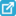View current table in a new windowDownload asVeiw figureFigures index
Figure 1. pH curve of ascorbic acid (0.1 M) against standardized NaOH (0.09 M)Download asVeiw figureFigures index
Figure 2. pH curve of malonic acid (0.05 M) against standardized NaOH(0.09M) [pKa1=2.85, pKa2= 5.70]Download asVeiw figureFigures index
Figure 3. pH curve of succinic acid (0.05M) against standardized NaOH (0.09 M)[pKa1= 4.21,pKa2= 5.64]Download asVeiw figureFigures index
Figure 4. pH curve of maleic acid (0.05 M) against standardized NaOH (0.09 M).[pKa1= 1.91, pKa2= 6.33]

Plot of an acid A has one inflection point, acid B has two inflection points, C has one and that of D has two inflection points (Figure 1 – Figure 4). From these curves it may appear that the unknown acid A is mono, B is di, C is mono, and that D is diprotic in nature. However, the reality is far from this assumption. We may improve our insight about these acid’s multiprotic behavior through an alternative method based upon equivalent weight calculation. This method involves the titration of an accurately weighed sample of the acid in question against strong base. If “n” is the number of protons transferred, then the true formula weight of the acid is an integral multiple of the calculated equivalent weight for monoprotic acid and twice as large as the equivalent weight for diprotic acid [FW = n x EW]. If we assume that titration involves the transfer of n protons then the moles of base needed to reach the equivalence point is given as

Moles titrant = n x moles analyte

And the formula weight is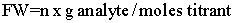The true formula weight is therefore an integral multiple of the calculated equivalent weight i.e.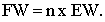From the pH curves for acid A, B, C, and D, final equivalence point volumes of strong base are noted and equivalent weights of these acids are calculated. If 0.4823g, 0.1405g, 0.1295g and 0.1537g of unknown acids A, B, C, and D are taken respectively, equivalents weights of acids and “n” can be calculated as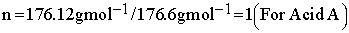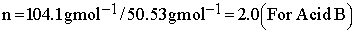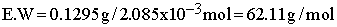From calculation of equivalent weights it is found that acid A is a monoprotic and acid B and D are diprotic. Experimentally developed pH curves of these acids also confirm the same as curve for acid A has only one inflection point and that two inflection points appear for acid B and D. However, in case of acid C, the formula weight of acid is found to be twice of the equivalent weight which shows that it is a diprotic acid but the curve has only one inflection point. First and second derivative of curve C (Figure 5, Figure 6) also show only one change. In spite of that C is a diprotic acid, its successive dissociation constants being closer in magnitude, it portrays only one inflection point, suggesting it to be monoprotic.Download asVeiw figureFigures index
Figure 5. First derivative curve of succinic acidDownload asVeiw figureFigures index
Figure 6. Second derivative curve of succinic acid

In case of a diprotic acid, the scenario is such that two distinct inflection points are observed when reaction (1) is essentially completed prior to reaction (2).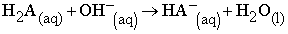(1)(2)

The successive acid dissociation constants of acid B and D differ significantly in magnitude (see caption of figures for values of acid dissociation constant), accordingly they show two inflection points on the curve. Contrary to that C is a diprotic acid but its successive dissociation constants are quite closer in magnitude so it highlights only one change. For these type of weak multiprotic acids pH curve may not be used to mark the step wise dissociation of an acid.

4. Conclusions

This study provides an alternate way for establishing multiprotic behavior of a weak acid. We proposed the equivalent weight calculation for those multiprotic weak acids whose inflection points are missed or not identified in the titration curves. This work would help to design a new laboratory exercise in which student may asked to predict the multiproticity of unknown weak acid from the pH curve and make it confirm by equivalent weight calculations.

References

  Harris, D. C., Quantitative Chemical Analysis, 3rd edition, Craig Bleyer Publisher W. H. Freeman and Company, New York, 2007.In article  Eastman, E. D., “Occurrence and Position of the principle Inflection Point in Certain Acid-Base Titration Curves”, J. Am. Chem. Soc., 56 (12), 2646-2648, Dec. 1934.In article View Article  Roller, P. S., “Theory of the End point in Electrometric Titrations”, J. Am. Chem. Soc., 50 (1), 1-8, Jan. 1928.In article View Article  Marinenko, G. and Champion, C. E. , “High precision Coulometric Titrations of Boric Acid”, J of Research of the National Bureau of Standards-A. Physics and Chemistry, 75 A (5), 421-428, Sep. 1971.In article  Harvey, D., Modern Analytical Chemistry, 1st edition, James M. Smith Publisher McGraw-Hill Companies, Inc., USA, 2000.In article